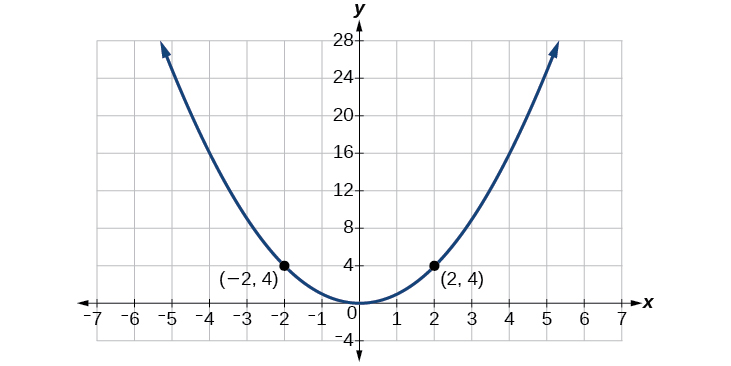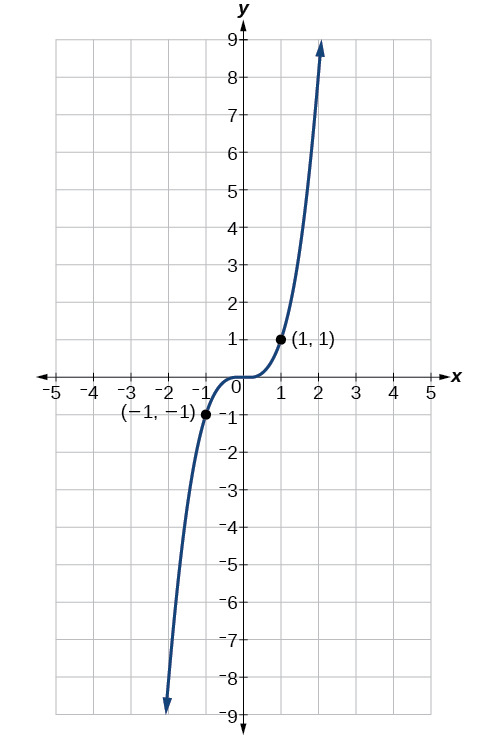# 7.4 The other trigonometric functions  (Page 3/14)

 Page 3 / 14

Use reference angles to find all six trigonometric functions of $\text{\hspace{0.17em}}-\frac{7\pi }{4}.$

$\begin{array}{l}\\ \mathrm{sin}\left(\frac{-7\pi }{4}\right)=\frac{\sqrt{2}}{2},\mathrm{cos}\left(\frac{-7\pi }{4}\right)=\frac{\sqrt{2}}{2},\mathrm{tan}\left(\frac{-7\pi }{4}\right)=1,\\ \mathrm{sec}\left(\frac{-7\pi }{4}\right)=\sqrt{2},\mathrm{csc}\left(\frac{-7\pi }{4}\right)=\sqrt{2},\mathrm{cot}\left(\frac{-7\pi }{4}\right)=1\end{array}$

## Using even and odd trigonometric functions

To be able to use our six trigonometric functions freely with both positive and negative angle inputs, we should examine how each function treats a negative input. As it turns out, there is an important difference among the functions in this regard.

Consider the function $\text{\hspace{0.17em}}f\left(x\right)={x}^{2},$ shown in [link] . The graph of the function is symmetrical about the y -axis. All along the curve, any two points with opposite x -values have the same function value. This matches the result of calculation: $\text{\hspace{0.17em}}{\left(4\right)}^{2}={\left(-4\right)}^{2},{\left(-5\right)}^{2}={\left(5\right)}^{2},$ and so on. So $\text{\hspace{0.17em}}f\left(x\right)={x}^{2}\text{\hspace{0.17em}}$ is an even function, a function such that two inputs that are opposites have the same output. That means $\text{\hspace{0.17em}}f\left(-x\right)=f\left(x\right).$The function   f ( x ) = x 2   is an even function.

Now consider the function $\text{\hspace{0.17em}}f\left(x\right)={x}^{3},$ shown in [link] . The graph is not symmetrical about the y -axis. All along the graph, any two points with opposite x -values also have opposite y -values. So $\text{\hspace{0.17em}}f\left(x\right)={x}^{3}\text{\hspace{0.17em}}$ is an odd function, one such that two inputs that are opposites have outputs that are also opposites. That means $\text{\hspace{0.17em}}f\left(-x\right)=-f\left(x\right).$The function   f ( x ) = x 3   is an odd function.

We can test whether a trigonometric function is even or odd by drawing a unit circle with a positive and a negative angle, as in [link] . The sine of the positive angle is $\text{\hspace{0.17em}}y.\text{\hspace{0.17em}}$ The sine of the negative angle is $\text{\hspace{0.17em}}-y.\text{\hspace{0.17em}}$ The sine function, then, is an odd function. We can test each of the six trigonometric functions in this fashion. The results are shown in [link] .

## Even and odd trigonometric functions

An even function is one in which $\text{\hspace{0.17em}}f\left(-x\right)=f\left(x\right).$

An odd function is one in which $\text{\hspace{0.17em}}f\left(-x\right)=-f\left(x\right).$

Cosine and secant are even:

Sine, tangent, cosecant, and cotangent are odd:

## Using even and odd properties of trigonometric functions

If the secant of angle $\text{\hspace{0.17em}}t\text{\hspace{0.17em}}$ is 2, what is the secant of $\text{\hspace{0.17em}}-t?$

Secant is an even function. The secant of an angle is the same as the secant of its opposite. So if the secant of angle $\text{\hspace{0.17em}}t\text{\hspace{0.17em}}$ is 2, the secant of $\text{\hspace{0.17em}}-t\text{\hspace{0.17em}}$ is also 2.

If the cotangent of angle $\text{\hspace{0.17em}}t\text{\hspace{0.17em}}$ is $\text{\hspace{0.17em}}\sqrt{3},$ what is the cotangent of $\text{\hspace{0.17em}}-t?$

$-\sqrt{3}$

## Recognizing and using fundamental identities

We have explored a number of properties of trigonometric functions. Now, we can take the relationships a step further, and derive some fundamental identities. Identities are statements that are true for all values of the input on which they are defined. Usually, identities can be derived from definitions and relationships we already know. For example, the Pythagorean Identity    we learned earlier was derived from the Pythagorean Theorem and the definitions of sine and cosine.

## Fundamental identities

We can derive some useful identities    from the six trigonometric functions. The other four trigonometric functions can be related back to the sine and cosine functions using these basic relationships:

$\mathrm{tan}\text{\hspace{0.17em}}t=\frac{\mathrm{sin}\text{\hspace{0.17em}}t}{\mathrm{cos}\text{\hspace{0.17em}}t}$
$\mathrm{sec}\text{\hspace{0.17em}}t=\frac{1}{\mathrm{cos}\text{\hspace{0.17em}}t}$
$\mathrm{csc}\text{\hspace{0.17em}}t=\frac{1}{\mathrm{sin}\text{\hspace{0.17em}}t}$
$\text{cot}\text{\hspace{0.17em}}t=\frac{1}{\text{tan}\text{\hspace{0.17em}}t}=\frac{\text{cos}\text{\hspace{0.17em}}t}{\text{sin}\text{\hspace{0.17em}}t}$

#### Questions & Answers

write down the polynomial function with root 1/3,2,-3 with solution
if A and B are subspaces of V prove that (A+B)/B=A/(A-B)
write down the value of each of the following in surd form a)cos(-65°) b)sin(-180°)c)tan(225°)d)tan(135°)
Prove that (sinA/1-cosA - 1-cosA/sinA) (cosA/1-sinA - 1-sinA/cosA) = 4
what is the answer to dividing negative index
In a triangle ABC prove that. (b+c)cosA+(c+a)cosB+(a+b)cisC=a+b+c.
give me the waec 2019 questions
the polar co-ordinate of the point (-1, -1)
prove the identites sin x ( 1+ tan x )+ cos x ( 1+ cot x )= sec x + cosec x
tanh`(x-iy) =A+iB, find A and B
B=Ai-itan(hx-hiy)
Rukmini
what is the addition of 101011 with 101010
If those numbers are binary, it's 1010101. If they are base 10, it's 202021.
Jack
extra power 4 minus 5 x cube + 7 x square minus 5 x + 1 equal to zero
the gradient function of a curve is 2x+4 and the curve passes through point (1,4) find the equation of the curve
1+cos²A/cos²A=2cosec²A-1
test for convergence the series 1+x/2+2!/9x3ByByBy Gerr ZenBy RhodesBy